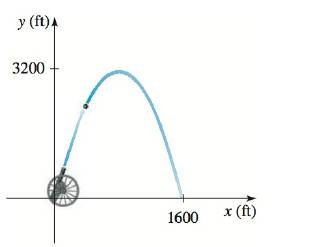Chapter 12.4, Problem 66E### Algebra and Trigonometry (MindTap ...

4th Edition
James Stewart + 2 others
ISBN: 9781305071742

#### Solutions

Chapter
Section### Algebra and Trigonometry (MindTap ...

4th Edition
James Stewart + 2 others
ISBN: 9781305071742
Textbook Problem

# Path of a Cannonball A cannon fires a cannonball as shown in the figure. The path of the cannonball is a parabola with vertex at the highest point of the path. If the cannonball lands 1600 ft from the cannon and the highest point it reaches is 3200 ft above the ground, find an equation for the path of the cannonball. Place the origin at the location of the cannon.To determine

The equation for the path of a cannonball.

Explanation

Given:

The graph of the path of a cannonball is given as

Approach:

The standard form of a parabola is given as

(xh)2=4p(yk) ...(1)

Calculation:

From the graph the vertex is (16002,3200) and the point (0,0) is on the graph. Then, it should satisfy (1).

Substitute h=800, k=3200, x=0 and y=0 in (1)

### Still sussing out bartleby?

Check out a sample textbook solution.

See a sample solution

#### The Solution to Your Study Problems

Bartleby provides explanations to thousands of textbook problems written by our experts, many with advanced degrees!

Get Started